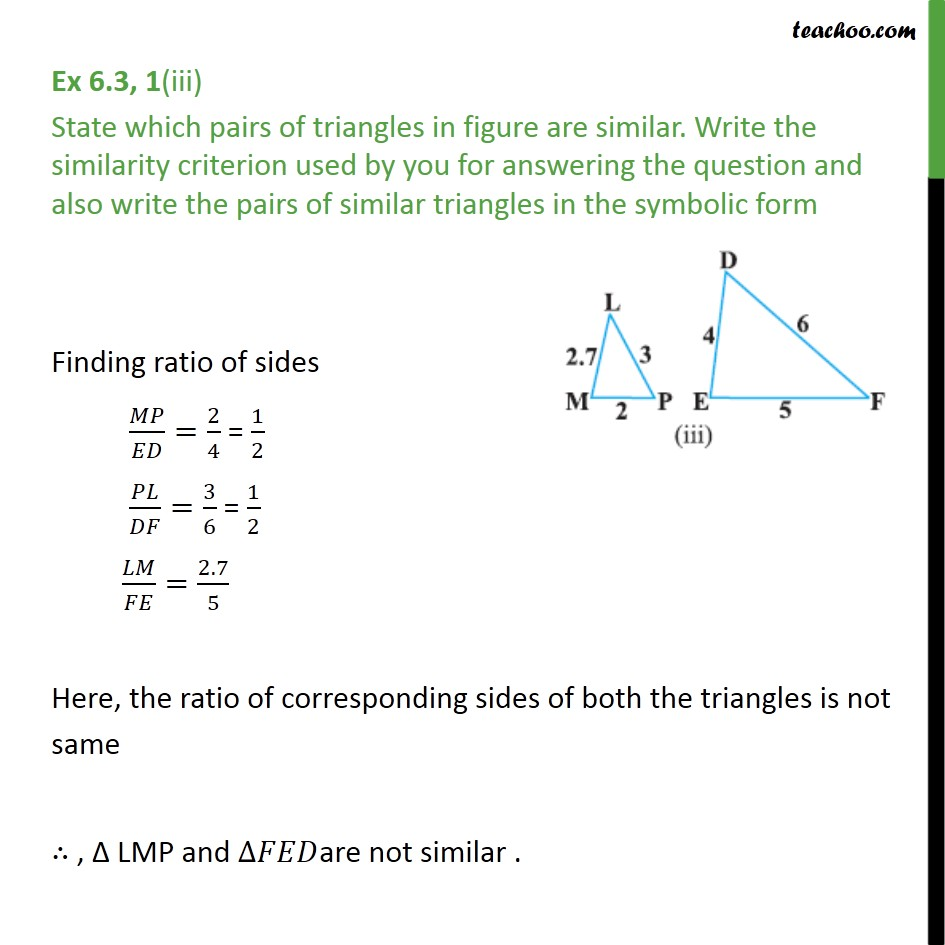Ex 6.3

Chapter 6 Class 10 Triangles
Serial order wiseLearn in your speed, with individual attention - Teachoo Maths 1-on-1 Class

### Transcript

Ex 6.3, 1(iii) State which pairs of triangles in figure are similar. Write the similarity criterion used by you for answering the question and also write the pairs of similar triangles in the symbolic form Finding ratio of sides / =2/4 = 1/2 / =3/6 = 1/2 / =2.7/5 Here, the ratio of corresponding sides of both the triangles is not same , LMP and are not similar .